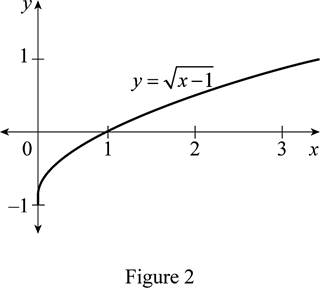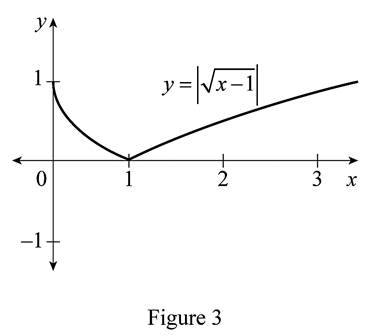# The function y = x by hand without plotting any points and then graph of the function y = | x − 1 | .### Single Variable Calculus: Concepts...

4th Edition
James Stewart
Publisher: Cengage Learning
ISBN: 9781337687805### Single Variable Calculus: Concepts...

4th Edition
James Stewart
Publisher: Cengage Learning
ISBN: 9781337687805

#### Solutions

Chapter 1.3, Problem 23E
To determine

## To sketch: The function y=x by hand without plotting any points and then graph of the function y=|x−1| .

Expert Solution

### Explanation of Solution

The standard graph of the function y=x is roughly drawn is shown below in Figure 1.Then, draw the graph of y=x1 by shifting the graph y=x by 1 unit downward. Thus, the graph of y=x1 is shown below in Figure 2.From Figure 2, it is observed that Figure 1 is shifted 1 unit to the downward. Then, draw the graph of y=|x1| , by reflecting the portion of the graph y=x1 that appeared below the x-axis about the origin. Thus, the graph of y=x1 is shown below in Figure 3.Observe that Figure 3is obtainedfrom Figure 1 in such a way that it is shifted1 unit downward and then reflected the curve that appear below the x-axis about the origin.

### Have a homework question?

Subscribe to bartleby learn! Ask subject matter experts 30 homework questions each month. Plus, you’ll have access to millions of step-by-step textbook answers!